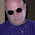## Plant Caterpillar Apple Puzzle - Can You Solve?

Explanation
Equation 1: 5 + 5 + 5 = 15 (Plant = 5; Note that the plant has 5 leaves)
Equation 2: 3 + 3 + 3 = 9 (Caterpillar = 3)
Equation 3: 9 + (9+3) + 9 = 30 (Apple = 9; Note that in the second apple's leaf, there is a caterpillar)
Equation 4: 3 x (6+3) - 12 = 15 (Note that the plant has 6 leaves, so plant = 6 & in one of the leaf there is a caterpillar, so (6+3)) (Also note that in the apple's leaf there is a caterpillar, so 12).

1.It’s wrong. It’s not 3+ but 3*

1.2.3.1.Primeiro q um número sobre o outro não se soma.
A pagar em cima da maçã equivale ao número 3 e a maçã o número 10 então é 10 elevado a 3 potência equivale a 1000.
Ou seja A resposta seria

3×6-1000= 982.

Que um professor(a) me corrija se eu estiver errado.

4.5.6.That's not how maths works.

7.It's 14 not 15 times the catapillar with 6 leaves, plus catapiller then take away the Apple and catapillar gets you 14

8.Ignore last message now it's defo 15 lol

9.10.This comment has been removed by the author.

11.I thought you first do any addition or subtraction before multiplication, no?

12.It is ridiculous. The answer can vary according to equation 3. Instead of:
9+(9+3)+9=30
We may write:
10+(7+3)+10=30
Which will ultimately result to:
3*(6+3)-10=17

13.Within this webpage, you'll see the page, you need to understand this data. chess